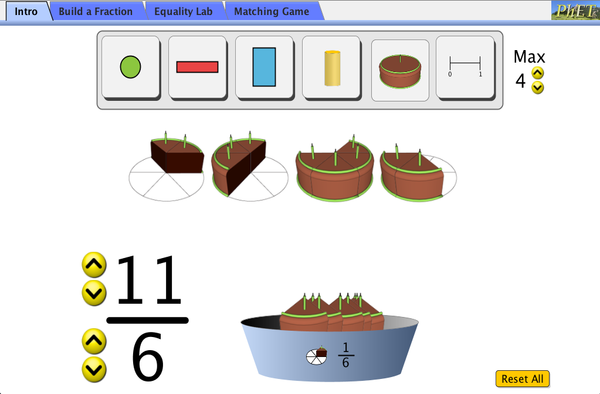# 分数介绍下载嵌入代码 关闭 插入一张图片，当点击这个图片时启动仿真程序。回到HTML5 版本

• 分数
• 等值分数
• 可约分数
• 数轴

### 示范 学习目标

• 预测和解释如何改变分数的分子来影响分数的值。
• 预测和解释如何改变分数的分母来影响分数的值。
• 在一个分数找、数字部分的一个图像和一个在数轴上点之间进行转换。
• 使用数字和图片出匹配的分数。
• 使用不同的数字使分数等值。
• 在不同的图像模式中匹配分数。
• 使用数字和图片匹配分数。

### 标准对齐

#### Common Core - Math

1.G.A.3
Partition circles and rectangles into two and four equal shares, describe the shares using the words halves, fourths, and quarters, and use the phrases half of, fourth of, and quarter of. Describe the whole as two of, or four of the shares. Understand for these examples that decomposing into more equal shares creates smaller shares.
2.G.A.2
Partition a rectangle into rows and columns of same-size squares and count to find the total number of them.
2.G.A.3
Partition circles and rectangles into two, three, or four equal shares, describe the shares using the words halves, thirds, half of, a third of, etc., and describe the whole as two halves, three thirds, four fourths. Recognize that equal shares of identical wholes need not have the same shape.
3.NF.A.1
Understand a fraction 1/b as the quantity formed by 1 part when a whole is partitioned into b equal parts; understand a fraction a/b as the quantity formed by a parts of size 1/b.
3.NF.A.2
Understand a fraction as a number on the number line; represent fractions on a number line diagram.
3.NF.A.2a
Represent a fraction 1/b on a number line diagram by defining the interval from 0 to 1 as the whole and partitioning it into b equal parts. Recognize that each part has size 1/b and that the endpoint of the part based at 0 locates the number 1/b on the number line.
3.NF.A.2b
Represent a fraction a/b on a number line diagram by marking off a lengths 1/b from 0. Recognize that the resulting interval has size a/b and that its endpoint locates the number a/b on the number line.
3.NF.A.3
Explain equivalence of fractions in special cases, and compare fractions by reasoning about their size.
3.NF.A.3a
Understand two fractions as equivalent (equal) if they are the same size, or the same point on a number line.
3.NF.A.3b
Recognize and generate simple equivalent fractions, e.g., 1/2 = 2/4, 4/6 = 2/3. Explain why the fractions are equivalent, e.g., by using a visual fraction model.
3.NF.A.3d
Compare two fractions with the same numerator or the same denominator by reasoning about their size. Recognize that comparisons are valid only when the two fractions refer to the same whole. Record the results of comparisons with the symbols >, =, or <, and justify the conclusions, e.g., by using a visual fraction model.

### 教师指南仿真程序控制概述,简化模型,学生视角的思考 ( PDF ).

### 教师提交的活动

What is a Fraction?Anonymous K-5 其它 数学
Comparing and Ordering Fractins Day 2 of 3Meaghan Hixson K-5 指导 数学
What is a Fraction? Day 1 of 3Meaghan Hixson K-5 指导 数学
Equivalent Fractions Day 3 of 3Meaghan Hixson K-5 指导 数学
Fractions 3 Day UnitMeaghan Hixson K-5 指导 数学
PRIMARIA: Alineación con programas de la SEP México (2011 y 2017)Diana López K-5

How do PhET simulations fit in my middle school program?Sarah Borenstein 初中 其它 化学

MS and HS TEK to Sim Alignment Elyse Zimmer 初中

분수 소개 SIM 사용설명서 이화국(Wha Kuk Lee) 初中 家庭作业

NGSS Simulation Alignment/Correlation Matthew Huffine 高中

K-5

Comparing Fractions with Like Numerators and Denominators Margaret McCabe 初中
K-5

Building Fractions Michellle Fiumefreddo K-5 概念性问题 数学

Windows Macintosh Linux
Microsoft Windows
XP/Vista/7/8.1/10